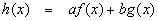Properties of Derivatives

This article covers laws that allow us to build up derivatives of complicated functions from simpler ones. These laws form part of the everyday tools of differential calculus.

Suppose f(x) and g(x) are differentiable functions and a and b are real numbers. Then the functionis differentiable and the derivative isLinear Combination Rule

This linearity law applies to many, many mathematical processes, so much so that we feel it is important to emphasize its nature with alternate notation.

Let us represent differentiation with respect to x by applying an operator Dx to a function:The last notation on the right is the older, traditional notation for derivative and is included for completeness. Using this notation, the linearity law becomesLinearity is so common that it behooves the reader to thoroughly digest this concept.

Find the derivative ofSolution

Using linearity,We can extend the notion of linearity to cover any number of constants and functions.

LetThenThis rule can be applied for any finite number of terms.

This rule is used to find the derivative of a product of two functions.

LetThenIn short,Definition of Product Rule

Use the product rule to find the derivative ofSolution

Here,ThusNote: Although this can be simplified, most professors (and graders!) prefer that you leave it as it is – that way, use of the product rule is clear. Also, astute readers probably observed that we could have more easily simplified h(x) algebraically first and then applied the linear combination rule. While that may be true for this example, in later sections, we will cover functions where the only practical way to do the derivative is by use of the product rule. In some cases, the easiest way to do a derivative is not the best way.

The quotient rule gives the derivative of one function divided by another. LetAnd require that g(x) not be zero. ThenIn short,Definition of the Quotient Rule

Find the derivative of the functionSolution

Here,So,As before, algebraic simplification is not always a good idea.

The chain rule is used in the case of a function of a function, also known as a composite function or as a composition of functions. There are two common notations with identical meanings:As with linear combinations, products and quotients, composition is another tool that allows us to build more complicated functions from simpler ones. Compostions are particularly useful in the case where a given problem has so-called chained dependencies where the quantity we wish to study is a function of a second quantity which in turn depends on a third quantity. These chained dependencies arise in practical applications so often they are given the special name related rates problems.

We start with two assumptions: g must be continuous and differentiable at x0 and f must be continuous and differentiable at g(x0). Using the slope form of the definition of the derivative:Now, if we defineThen by the continuity of g(x),Put another way,This allows us to make the substitutionNow we haveThis is the chain rule:Definition of the Chain Rule (function of a function version)

Using the composition notation givesDefinition of the Chain Rule (composition version)

Find the derivative ofSolution

We need to define the inside (g(x)) and outside (f(x)) functions:Differentiating each givesNow we apply the chain ruleClearly, this example would have been very difficult without the chain rule - any attempt to multiply out the polynomial would take far too long and would be very likely to produce math errors.

Recommended Books

The classic calculus problem book - very light on theory, plenty of problems with full solutions, more problems with answers

A simplified and updated version of the classic Schaum's Outline. Not as complete as the previous book, but enough for most students